# Dynamic Programming Introduction

Problem Statement:  Introduction To Dynamic Programming

In this article, we will be going to understand the concept of dynamic programming.

Dynamic Programming can be described as storing answers to various sub-problems to be used later whenever required to solve the main problem.

The two common dynamic programming approaches are:

• Memoization: Known as the “top-down” dynamic programming, usually the problem is solved in the direction of the main problem to the base cases.
• Tabulation: Known as the “bottom-up ” dynamic programming, usually the problem is solved in the direction of solving the base cases to the main problem

Note: The base case does not always mean smaller input. It depends upon the implementation of the algorithm.

The flow of this article will be as follows: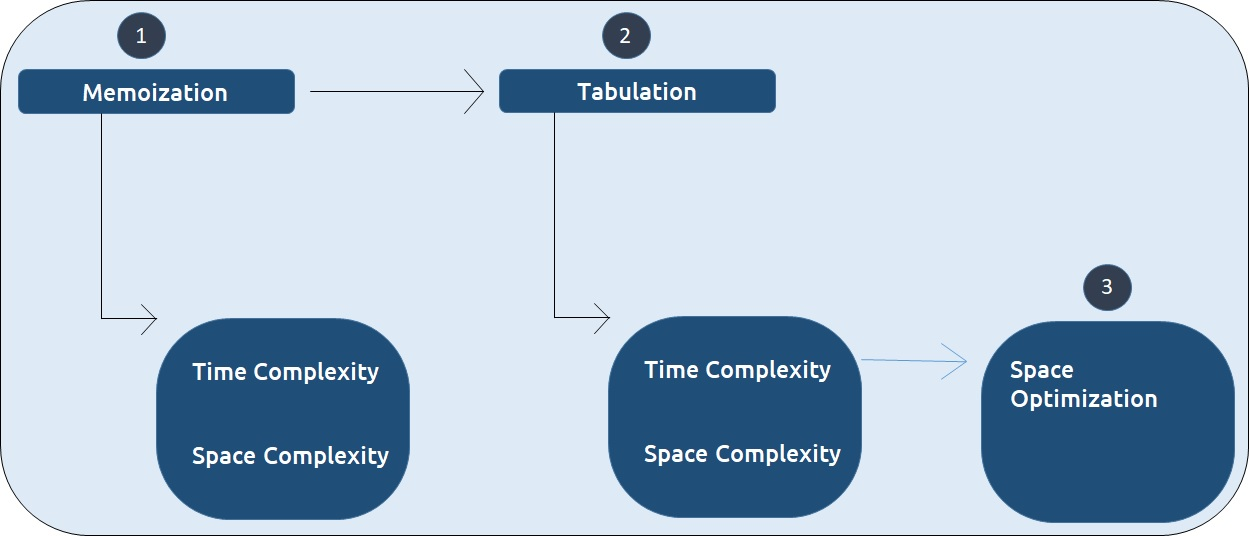We will be using the example of Fibonacci numbers here. The following series is called the Fibonacci series:

0,1,1,2,3,5,8,13,21,…

We need to find the nth Fibonacci number, where n is based on a 0-based index.

Every ith number of the series is equal to the sum of (i-1)th and (i-2)th number where the first and second number is given as 0 and 1 respectively.

Disclaimer: Don’t jump directly to the solution, try it out yourself first.

Pre-req: Recursion

### Solution :

Part – 1: Memoizaton

As every number is equal to the sum of the previous two terms, the recurrence relation can be written as: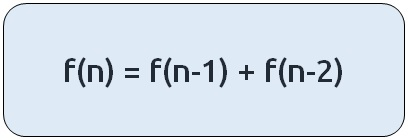The basic pseudo-code for the problem will be given as: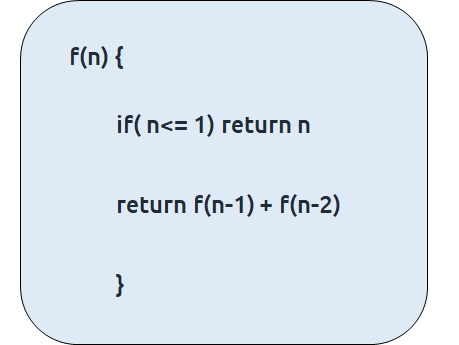If we draw the recursive tree for n=5, it will be: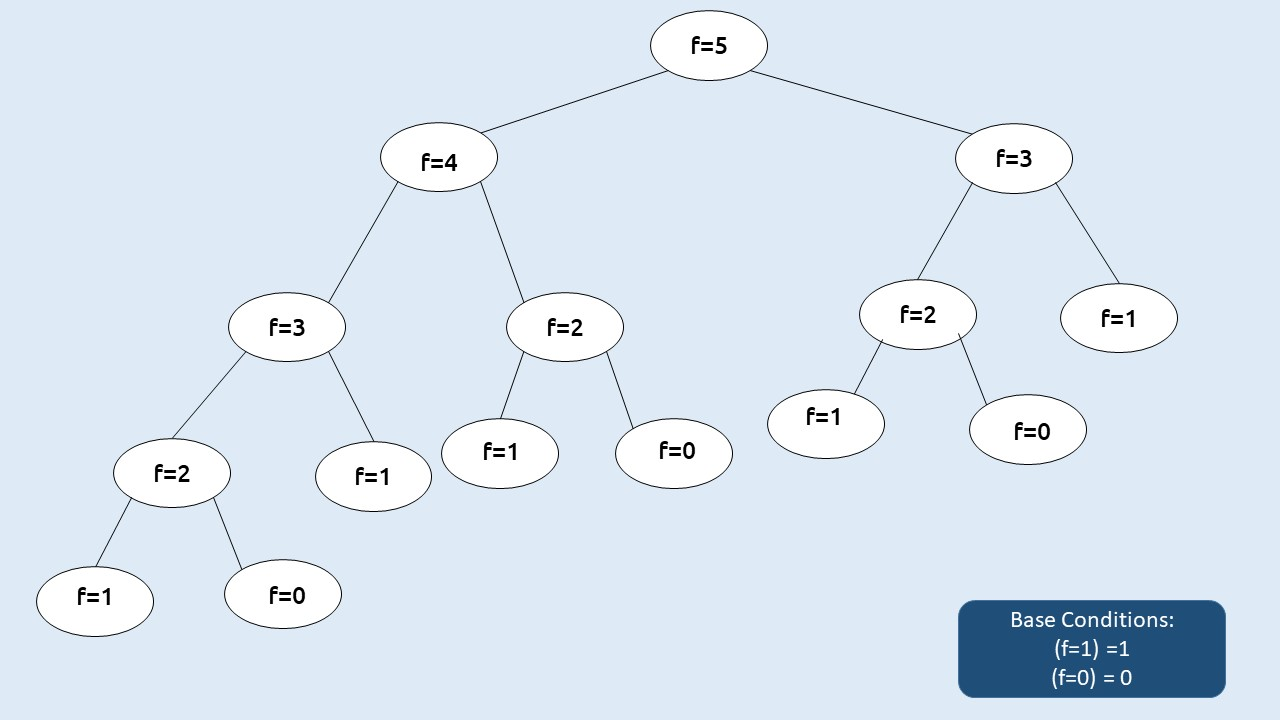If there are two recursive calls inside a function, the program will run the first call, finish its execution and then run the second call. Due to this reason, each and every call in the recursive tree will be executed. This gives the recursive code its exponential time complexity.
Can we improve on this? The answer is Yes!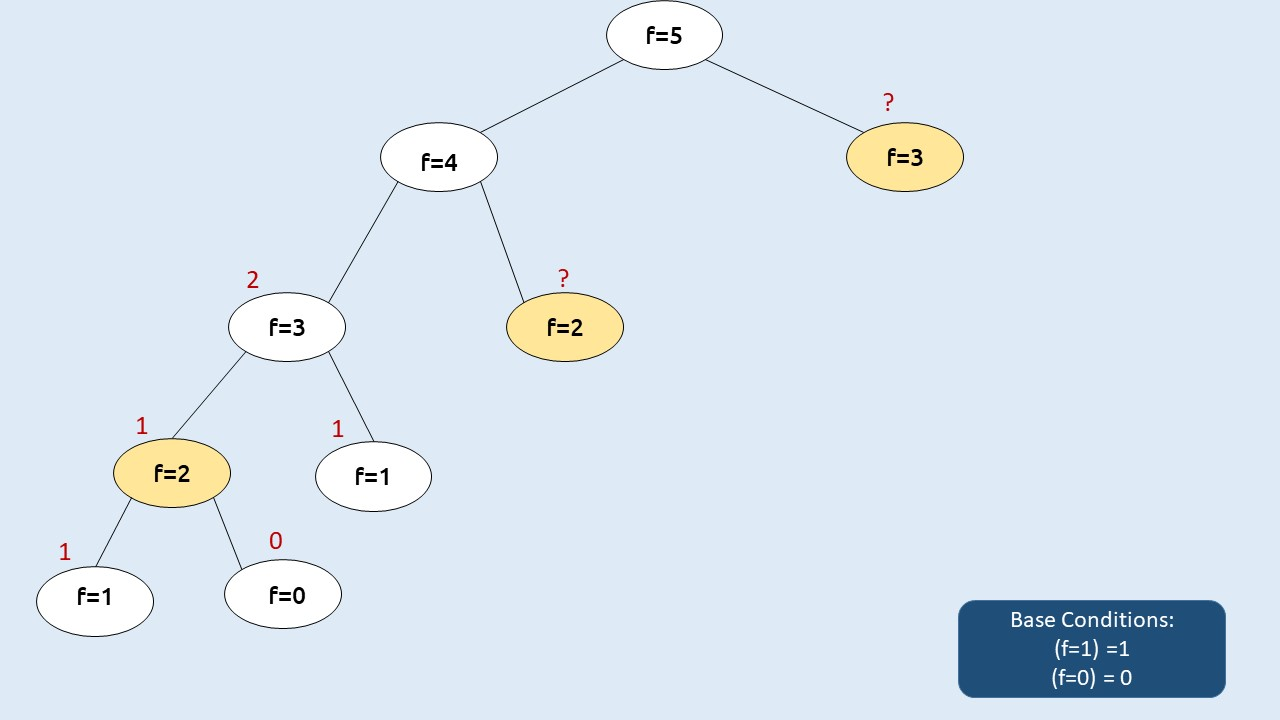We want to compute f(2) as the second call from f(4), but in the recursive tree we had already computed f(2) once (in the first recursive call of f(3) ) Similar is the case with f(3), therefore if we somehow store these values, the first time we calculated it then we can simply find its value in constant time whenever we need it. This technique is called Memoization. Here the cases marked in yellow are called overlapping sub-problems and we need to solve them only once during the code execution.

Steps to memoize a recursive solution:

Any recursive solution to a problem can be memoized using these three steps:

1. Create a dp[n+1] array initialized to -1.
2. Whenever we want to find the answer of a particular value (say n), we first check whether the answer is already calculated using the dp array(i.e dp[n]!= -1 ). If yes, simply return the value from the dp array.
3. If not, then we are finding the answer for the given value for the first time, we will use the recursive relation as usual but before returning from the function, we will set dp[n] to the solution we get.

Dry Run:

Declare a dp[] array of size n+1 and initialize it to -1.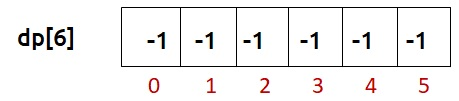Now, following the recursive code we see that at n=5, the value of dp is equal to -1 therefore we need to compute its value and go to the inner recursive calls. Similar is the case at n=4, n=3, and n=2. For n=2, we hit our two base conditions as the inner recursive calls.

As we traverse back after solving n=2, we update its dp array value to 1 ( the answer we got). Similarly, for the second recursive call for n=3, we again hit a base condition and we get an answer of f(n=3) as 2, we again update the dp array.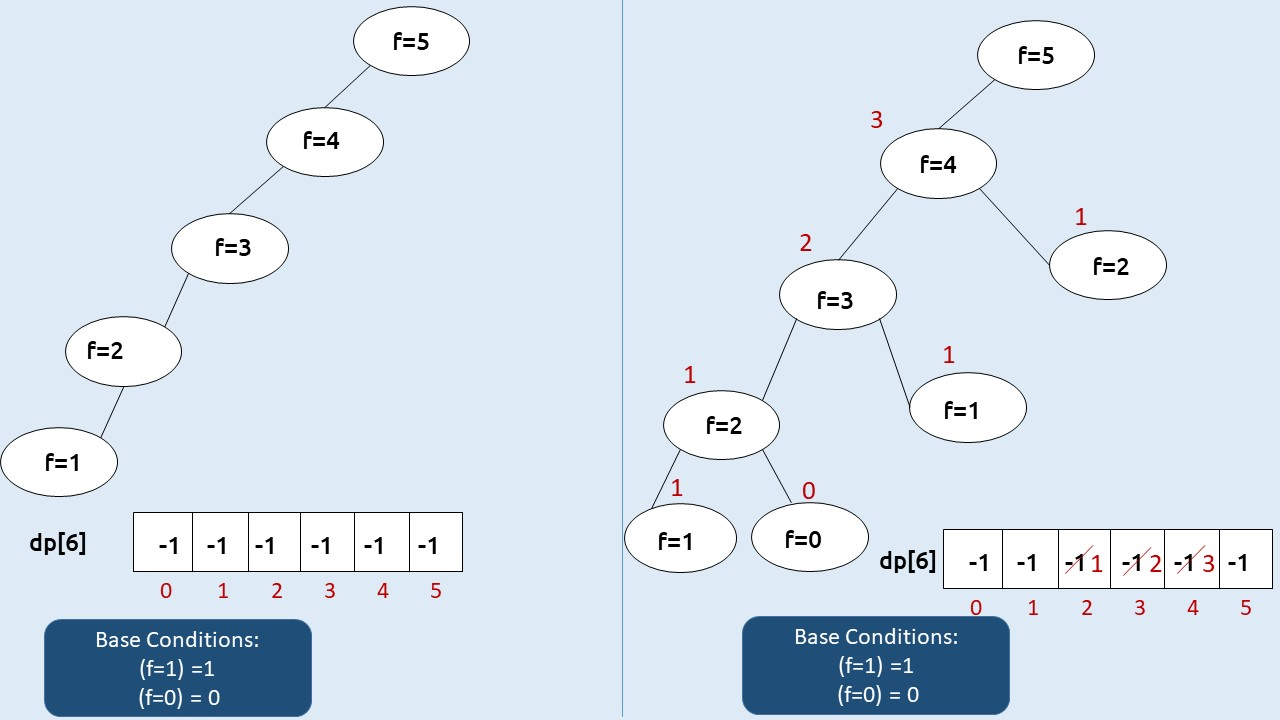Then for the second recursive call f(n=4), we see that dp is not equal to -1, which means that we have already solved this subproblem and we simply return the value at dp as our answer. Hence we get the answer of f(n=4) as 3(2+1).

Similarly, for the second recursive call f(n=5), we get dp as 2. Then we compute for(n=5) as 5(2+3).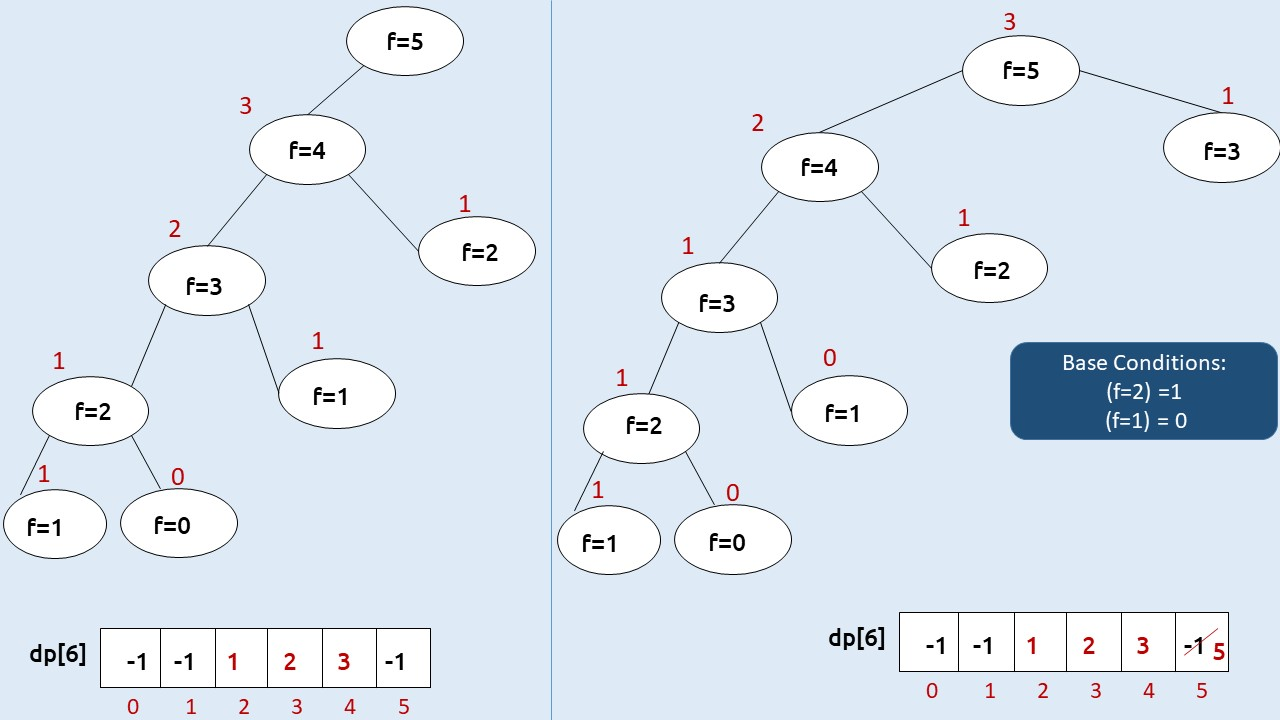Code:

## C++ Code

``````#include <bits/stdc++.h>

using namespace std;

int f(int n, vector<int>& dp){
if(n<=1) return n;

if(dp[n]!= -1) return dp[n];
return dp[n]= f(n-1,dp) + f(n-2,dp);
}

int main() {

int n=5;
vector<int> dp(n+1,-1);
cout<<f(n,dp);
return 0;
}
``````

Output: 5

Time Complexity: O(N)

Reason: The overlapping subproblems will return the answer in constant time O(1). Therefore the total number of new subproblems we solve is ‘n’. Hence total time complexity is O(N).

Space Complexity: O(N)

Reason: We are using a recursion stack space(O(N)) and an array (again O(N)). Therefore total space complexity will be O(N) + O(N) ≈ O(N)

## Java Code

``````import java.util.*;
class TUF{
static int f(int n, int[] dp){
if(n<=1) return n;

if(dp[n]!= -1) return dp[n];
return dp[n]= f(n-1,dp) + f(n-2,dp);
}

public static void main(String args[]) {

int n=5;
int dp[]=new int[n+1];
Arrays.fill(dp,-1);
System.out.println(f(n,dp));

}
}``````

Output: 5

Time Complexity: O(N)

Reason: The overlapping subproblems will return the answer in constant time O(1). Therefore the total number of new subproblems we solve is ‘n’. Hence total time complexity is O(N).

Space Complexity: O(N)

Reason: We are using a recursion stack space(O(N)) and an array (again O(N)). Therefore total space complexity will be O(N) + O(N) ≈ O(N)

Part -2: Tabulation

Tabulation is a ‘bottom-up’ approach where we start from the base case and reach the final answer that we want.

Steps to convert Recursive Solution to Tabulation one.

• Declare a dp[] array of size n+1.
• First initialize the base condition values, i.e i=0 and i=1 of the dp array as 0 and 1 respectively.
• Set an iterative loop which traverses the array( from index 2 to n) and for every index set its value as dp[i-1] + dp[i-2].

Code:

## C++ Code

``````#include <bits/stdc++.h>

using namespace std;

int main() {

int n=5;
vector<int> dp(n+1,-1);

dp= 0;
dp= 1;

for(int i=2; i<=n; i++){
dp[i] = dp[i-1]+ dp[i-2];
}
cout<<dp[n];
return 0;
}
``````

Output: 5

Time Complexity: O(N)

Reason: We are running a simple iterative loop

Space Complexity: O(N)

Reason: We are using an external array of size ‘n+1’.

## Java Code

``````import java.util.*;
class TUF{
public static void main(String args[]) {

int n=5;
int dp[]=new int[n+1];
Arrays.fill(dp,-1);
dp= 0;
dp= 1;

for(int i=2; i<=n; i++){
dp[i] = dp[i-1]+ dp[i-2];
}
System.out.println(dp[n]);

}
}``````

Output: 5

Time Complexity: O(N)

Reason: We are running a simple iterative loop

Space Complexity: O(N)

Reason: We are using an external array of size ‘n+1’.

Part 3: Space Optimization

If we closely look the relation,

dp[i] =  dp[i-1] + dp[i-2]

we see that for any i, we do need only the last two values in the array. So is there a need to maintain a whole array for it?

The answer is ‘No’. Let us call dp[i-1] as prev and dp[i-2] as prev2. Now understand the following illustration.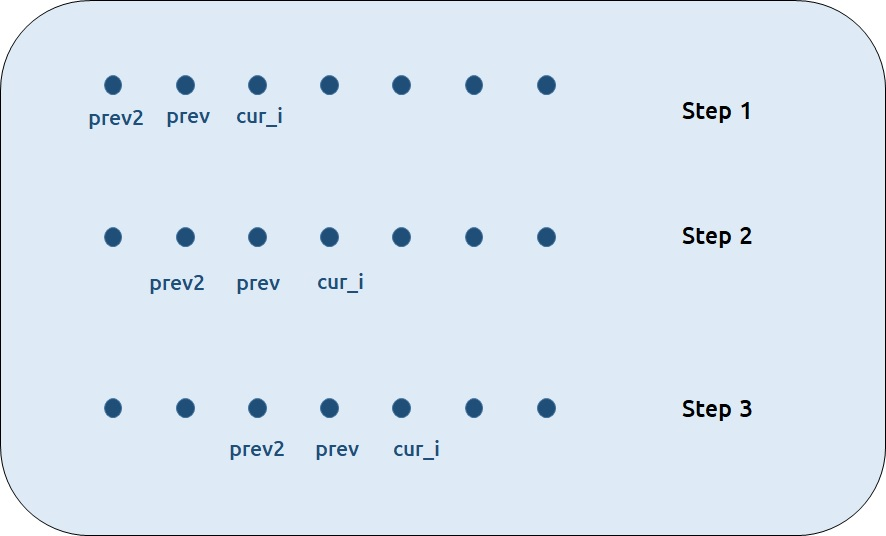• Each iteration’s cur_i and prev becomes the next iteration’s prev and prev2 respectively.
• Therefore after calculating cur_i, if we update prev and prev2 according to the next step, we will always get the answer.
• After the iterative loop has ended we can simply return prev as our answer.

Code:

## C++ Code

``````#include <bits/stdc++.h>

using namespace std;

int main() {

int n=5;

int prev2 = 0;
int prev = 1;

for(int i=2; i<=n; i++){
int cur_i = prev2+ prev;
prev2 = prev;
prev= cur_i;
}
cout<<prev;
return 0;
}

``````

Output: 5

Time Complexity: O(N)

Reason: We are running a simple iterative loop

Space Complexity: O(1)

Reason: We are not using any extra space

## Java Code

``````import java.util.*;
class TUF{
public static void main(String args[]) {
int n=5;

int prev2 = 0;
int prev = 1;

for(int i=2; i<=n; i++){
int cur_i = prev2+ prev;
prev2 = prev;
prev= cur_i;
}
System.out.println(prev);

}
}``````

Output: 5

Time Complexity: O(N)

Reason: We are running a simple iterative loop

Space Complexity: O(1)

Reason: We are not using any extra space

Special thanks to Anshuman Sharma for contributing to this article on takeUforward. If you also wish to share your knowledge with the takeUforward fam, please check out this article